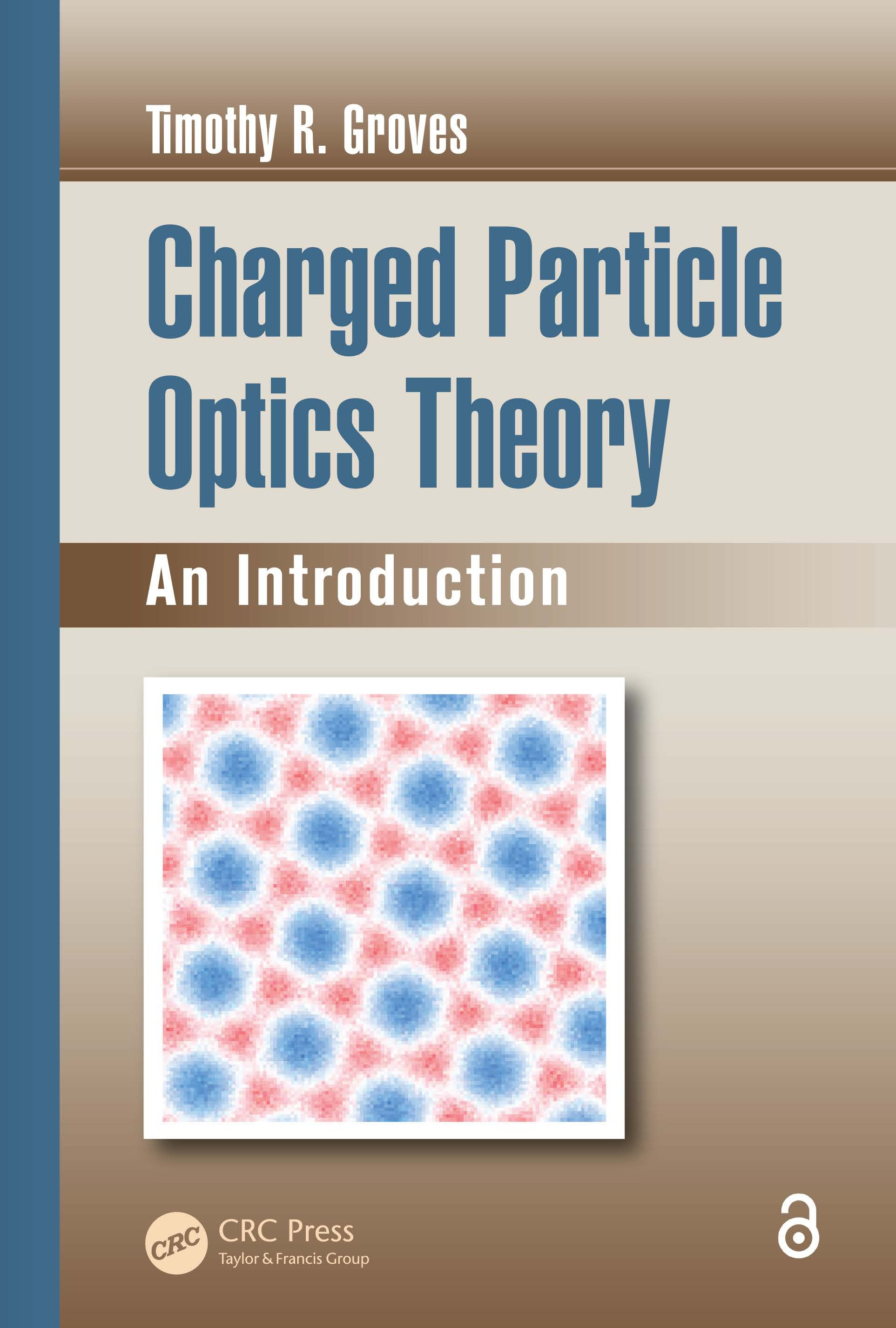# Charged Particle Optics Theory

## An Introduction, 1st Edition

CRC Press

369 pages

Paperback: 9780367377960
pub: 2019-08-30
SAVE ~\$14.99
Available for pre-order
\$74.95
\$59.96
x
Hardback: 9781482229943
pub: 2014-12-15
SAVE ~\$34.00
\$170.00
\$136.00
x
eBook (VitalSource) : 9781315215310
pub: 2017-12-19
from \$102.00

FREE Standard Shipping!

### Description

Charged Particle Optics Theory: An Introduction identifies the most important concepts of charged particle optics theory, and derives each mathematically from the first principles of physics. Assuming an advanced undergraduate-level understanding of calculus, this book follows a logical progression, with each concept building upon the preceding one. Beginning witha non-mathematical survey of the optical nature of a charged particle beam, the text:

• Discusses both geometrical and wave optics, as well as the correspondence between them

• Describes the two-body scattering problem, which is essential to the interaction of a fast charged particle with matter

• Introduces electron emission as a practical consequence of quantum mechanics

• Addresses the Fourier transform and the linear second-order differential equation

• Includes problems to amplify and fill in the theoretical details, with solutions presented separately

Charged Particle Optics Theory: An Introduction makes an ideal textbook as well as a convenient reference on the theoretical origins of the optics of charged particle beams. It is intended to prepare the reader to understand the large body of published research in this mature field, with the end result translated immediately to practical application.

Introduction: The Optical Nature of a Charged Particle Beam. Geometrical Optics. Wave Optics. Particle Scattering. Electron Emission from Solids. Appendix A: The Fourier Transform. Appendix B: Linear Second-Order Differential Equation.